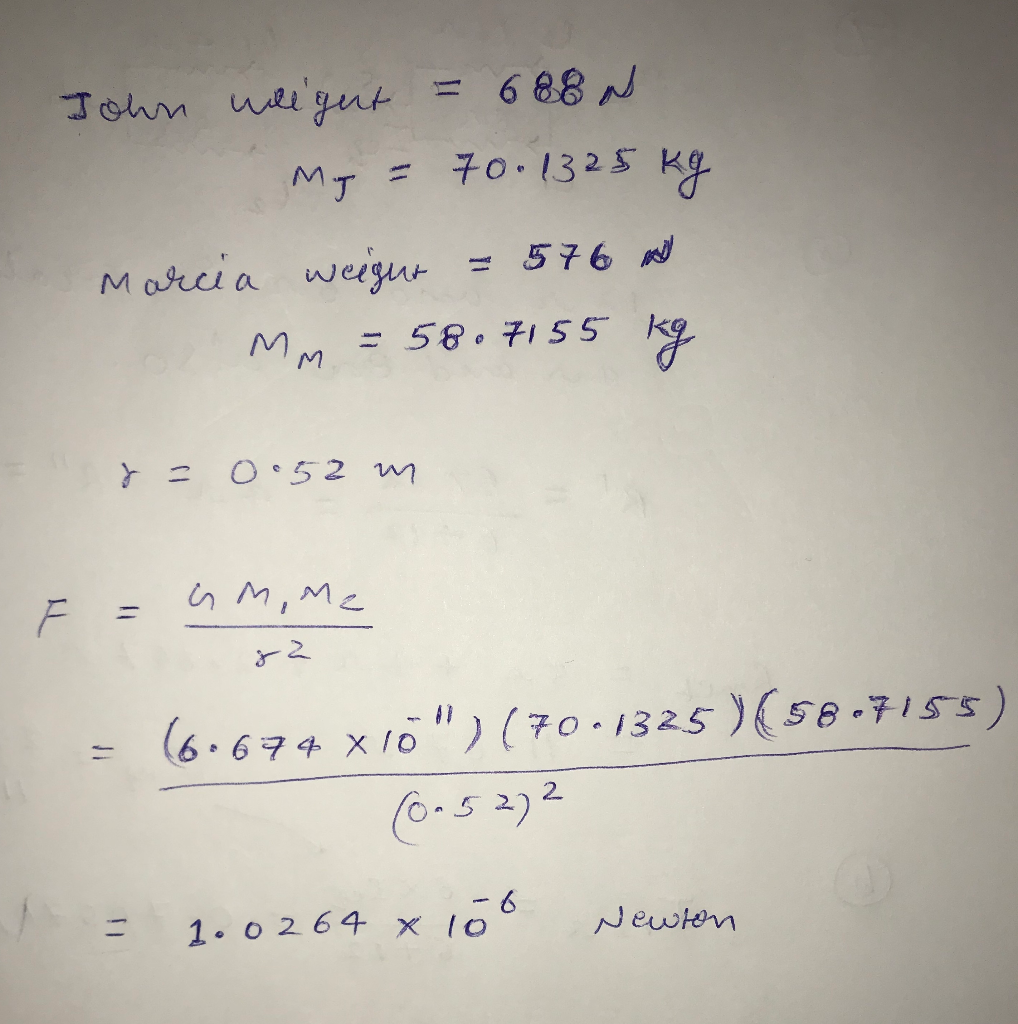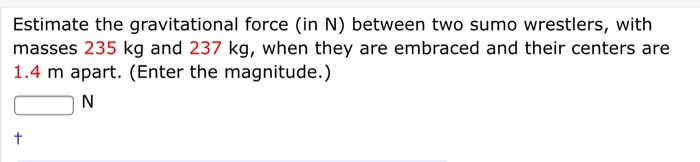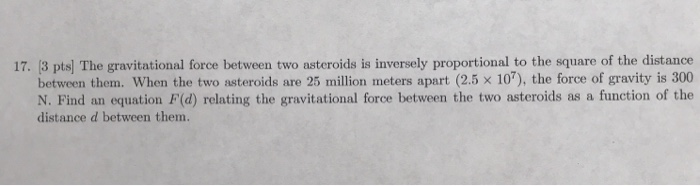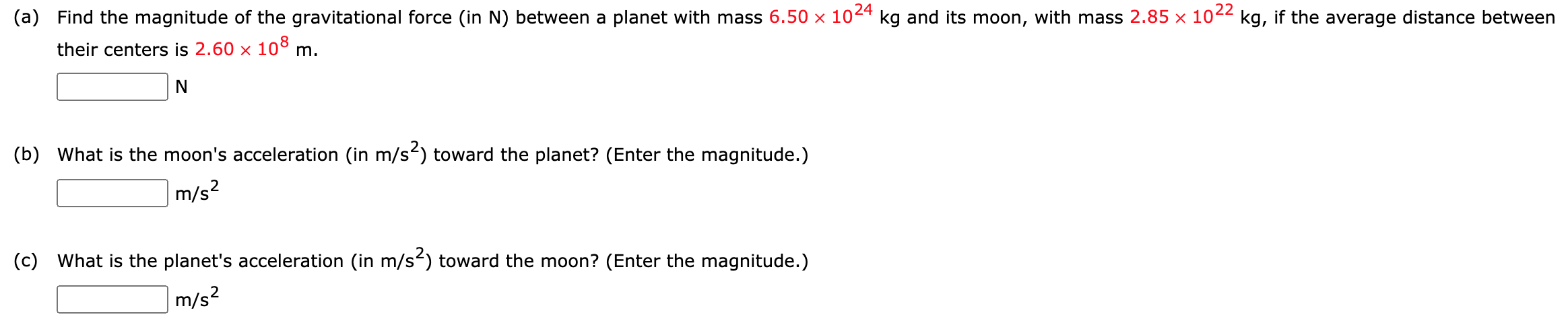Question

John weighs 688 N, and Marcia weighs 576 N. Estimate the gravitational force between them when they are 0.52 m apart.#### Earn Coins

Coins can be redeemed for fabulous gifts.

Similar Homework Help Questions
• ### Estimate the gravitational force (in N) between two sumo wrestlers, with masses 235 kg and 237...Estimate the gravitational force (in N) between two sumo wrestlers, with masses 235 kg and 237 kg, when they are embraced and their centers are 1.4 m apart. (Enter the magnitude.) ON

• ### 17. (3 pts) The gravitational force between two asteroids is inversely proportional to the square of...17. (3 pts) The gravitational force between two asteroids is inversely proportional to the square of the distance between them. When the two asteroids are 25 million meters apart (2.5 10), the force of gravity is 300 N. Find an equation F(d) relating the gravitational force between the two asteroids as a function of the distance d between them.

• ### The gravitational force between two electrons 1.09 m apart is 4.56 10-71 N

The gravitational force between two electrons 1.09 m apart is 4.56 10-71 N. Find the mass of an electron. (Use G = 6.67 10-11 Nm2/kg2.)

• ### 2 balls have a mass of 0.838kg each. The gravitational force between them is 8.08e-11N. The...

2 balls have a mass of 0.838kg each. The gravitational force between them is 8.08e-11N. The universal constant G = 6.673e-11 Nm^2/kg^2. How far apart are the balls?

• ### What is the approximate value of the gravitational force between a 72 kg astronaut and a...

What is the approximate value of the gravitational force between a 72 kg astronaut and a 7.8 metric ton spacecraft when they are 72.5 m apart?

• ### Two bodies are exerting a gravitational force of 100N on each other. If the mass of each body doubles and the distance between them stays the same, what is the new gravitational force between them?

Two bodies are exerting a gravitational force of 100N on each other. If the mass of each body doubles and the distance between them stays the same, what is the new gravitational force between them?

• ### the force of gravity between two masses is 26 N when they are 7.5 apart. the...

the force of gravity between two masses is 26 N when they are 7.5 apart. the distance between them is changed and the force increases to 71 N. how far apart are they now?

• ### If the gravitational force is 12663 N between a satelite with a mass of 11 x...

If the gravitational force is 12663 N between a satelite with a mass of 11 x 10^2 kg and a planet with a mass of 2 x 10^20 kg, find the distance between them

• ### Find the magnitude of the gravitational force (in N) between a planet with mass 6.50 ✕...Find the magnitude of the gravitational force (in N) between a planet with mass 6.50 ✕ 1024 kg and its moon, with mass 2.85 ✕ 1022 kg, if the average distance between their centers is 2.60 ✕ 108 m. (a) Find the magnitude of the gravitational force (in N) between a planet with mass 6.50 x 1024 kg and its moon, with mass 2.85 x 1022 kg, if the average distance between their centers is 2.60 x 108 m. N...

• ### If the gravitational force between the electron (mass 9.11*10^-31 kg) and the proton(mass 1.67...

If the gravitational force between the electron (mass 9.11*10^-31 kg) and the proton(mass 1.67 *10^-27 kg) in a hydrogen atom is 5*10^-48N, how far apart are the twoparticles? The universal gravitational constant is 6.673*10^-11 N*m^2 / kg^2. answer in units of m